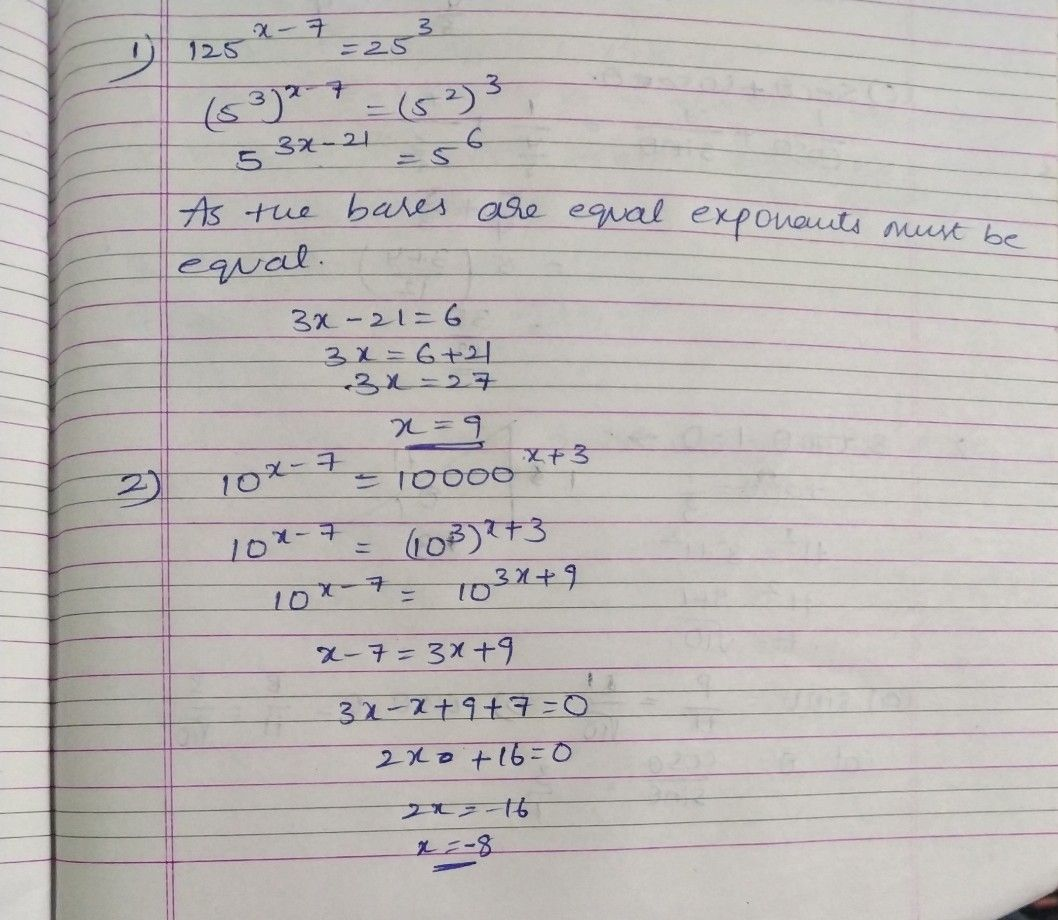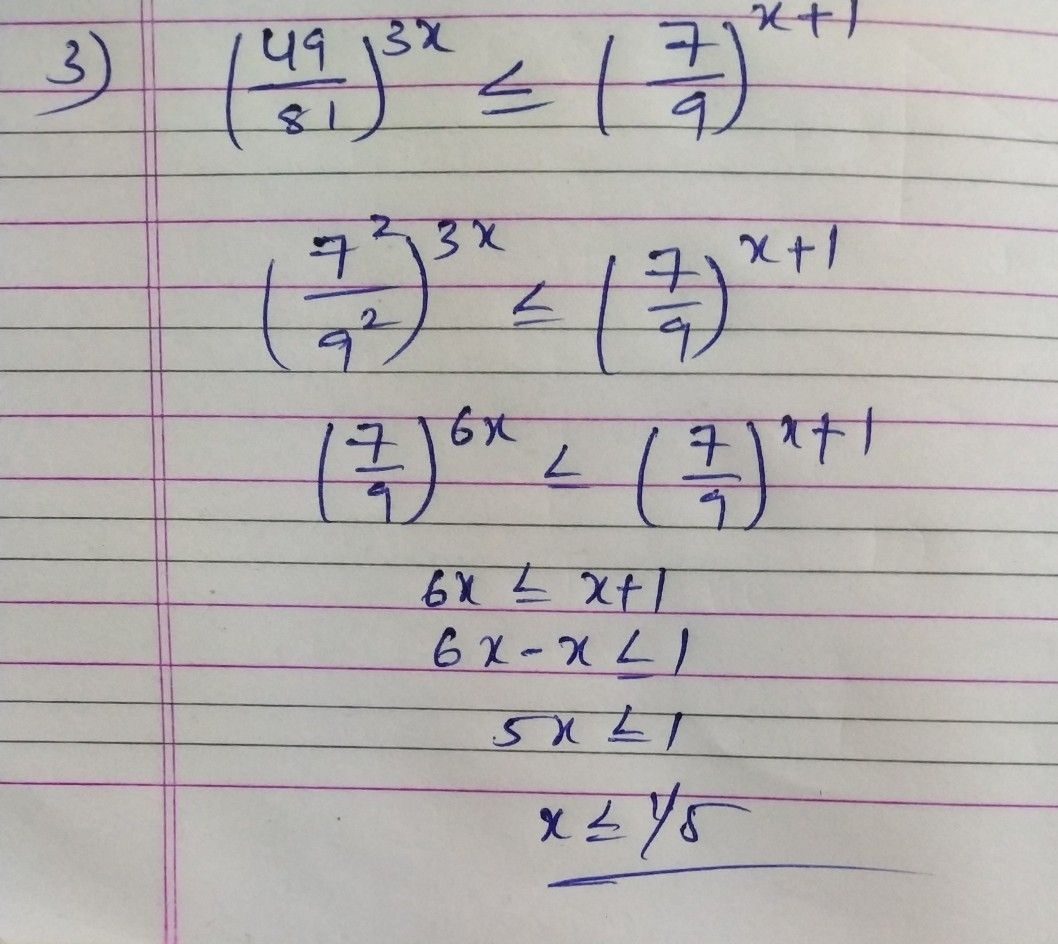Symbol
ProblemDetermine the value of x.. $1$ $125^{x-7}=25^{3}$ $10^{x-7}=10000^{x+3}$ $2.10^{x-7}$ $3$ $\left(\dfrac {49} {81}\right)^{3x}$ $\leq \left(\dfrac {7} {9}\right)^{x+1}$
10th-13th grade
Other
Search count: 106
SolutionQanda teacher - Raji